Question

# Consider the closed loop system a) Design a PD controller (that is, calculate K1 and K2) such that the system is stable and the steady-state error for the input r (t) = unity ramp let less than or equal to 0.02. b) Select a value from K1 and K2 and build

Consider the closed loop system

a) Design a PD controller (that is, calculate K1 and K2) such that the system is

stable and the steady-state error for the input r (t) = unity ramp let

less than or equal to 0.02.

b) Select a value from K1 and K2 and build the model in Simulink or solution

analytical to obtain the response of the system to the magnitude ramp

r (t) = 2t.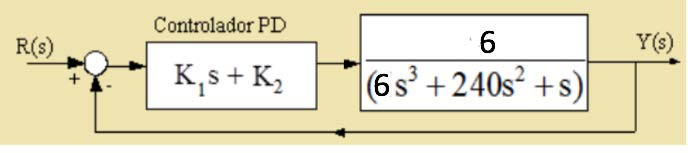We need at least 10 more requests to produce the answer.

0 / 10 have requested this problem solution

The more requests, the faster the answer.

All students who have requested the answer will be notified once they are available.

#### Earn Coins

Coins can be redeemed for fabulous gifts.

Similar Homework Help Questions
• ### PD & PID controller design Consider a unity feedback system with open loop transfer function, G(s)...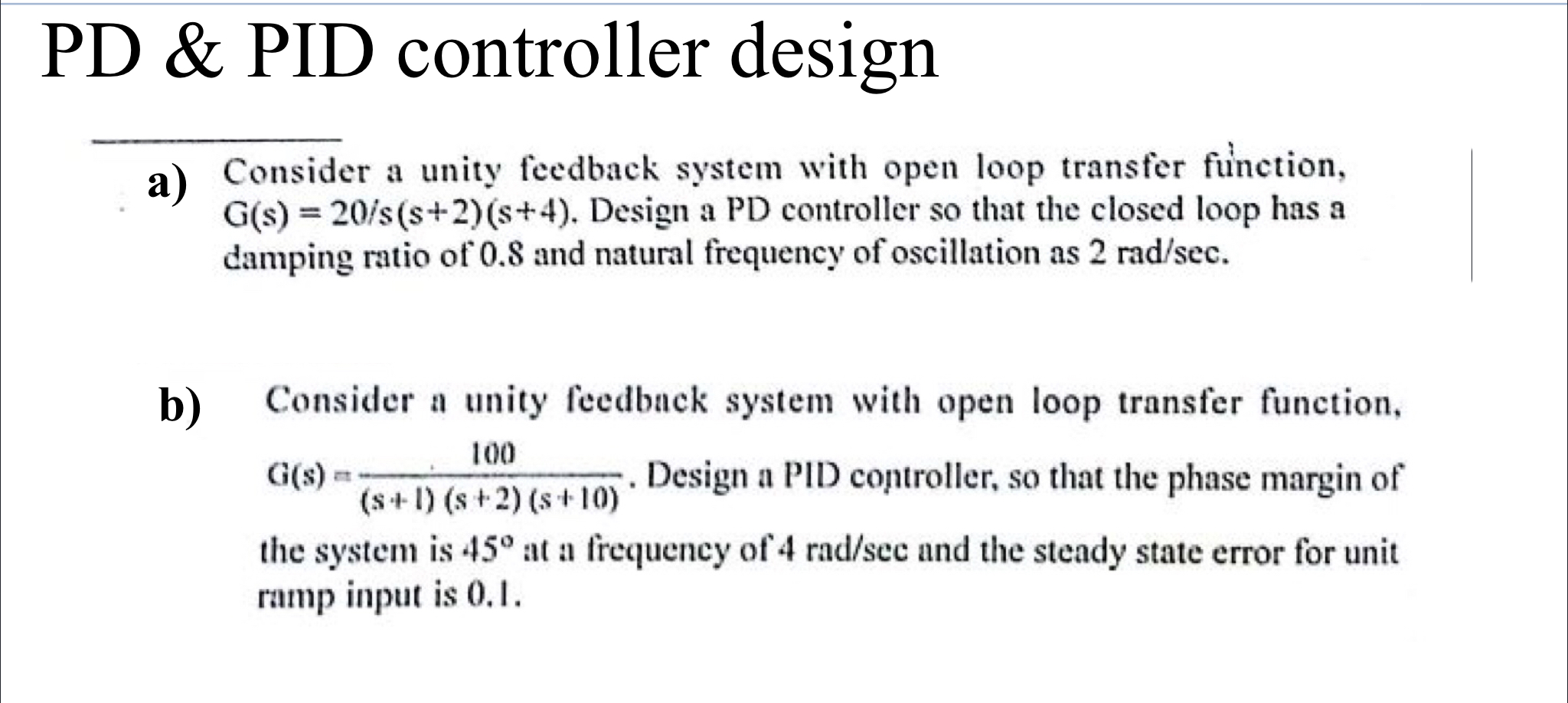PD & PID controller design Consider a unity feedback system with open loop transfer function, G(s) = 20/s(s+2)(8+4). Design a PD controller so that the closed loop has a damping ratio of 0.8 and natural frequency of oscillation as 2 rad/sec. b) 100 Consider a unity feedback system with open loop transfer function, aus. Design a PID controller, so that the phase margin of (S-1) (s + 2) (s+10) the system is 45° at a frequency of 4 rad/scc and...

• ### please solve as matlab code. The system in Figure 3 comprises a motor and a contoller. The performance requirements entail a steady state error for ramp input r(t) Ct, smaller than 0.01C. Here, C...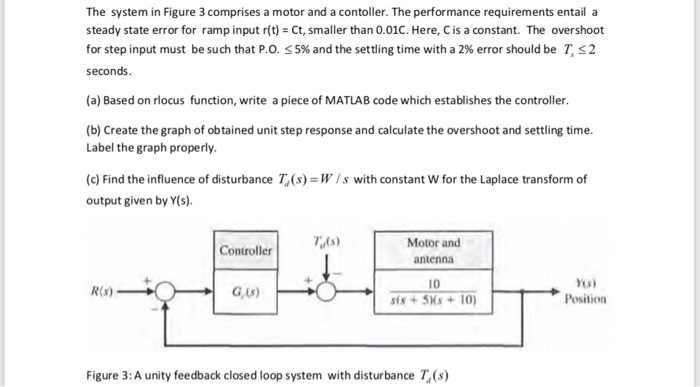please solve as matlab code. The system in Figure 3 comprises a motor and a contoller. The performance requirements entail a steady state error for ramp input r(t) Ct, smaller than 0.01C. Here, C is a constant. The overshoot for step input must be such that P.0. 5% and the settling time with a 2% error should be T, 2 seconds (a) Based on rlocus function, write a piece of MATLAB code which establishes the controller. (b) Create the graph...

• ### PROBLEM 4 A unity feedback closed loop control system is displayed in Figure 4 (a) Assume that the controller is given by G (s)-2. Based on the lsim function of MATLAB, calculate and obtain the g...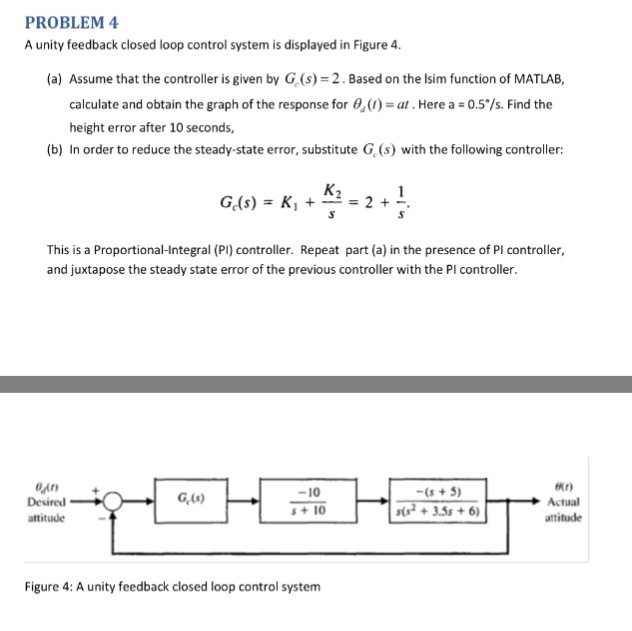PROBLEM 4 A unity feedback closed loop control system is displayed in Figure 4 (a) Assume that the controller is given by G (s)-2. Based on the lsim function of MATLAB, calculate and obtain the graph of the response for 0,(1)-a. Here a ; 0.5%, Find the height error after 10 seconds, (b) In order to reduce the steady-state error, substitute G. (s) with the following controller: K2 This is a Proportional-Integral (PI) controller. Repeat part (a) in the presence...

• ### Write as MATLAB code with comments thank you. The system in Figure 3 comprises a motor and a contoller. The performance requirements entail a steady state error for ramp input r(t) Ct, smaller than 0...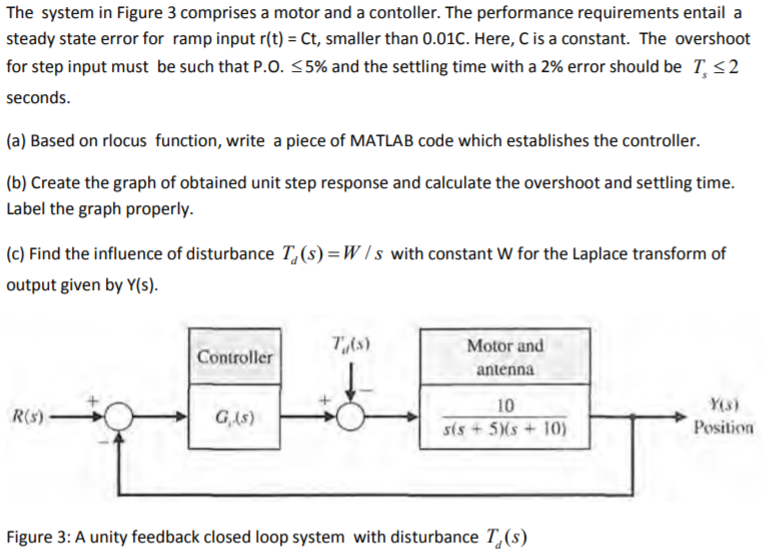Write as MATLAB code with comments thank you. The system in Figure 3 comprises a motor and a contoller. The performance requirements entail a steady state error for ramp input r(t) Ct, smaller than 0.01C. Here, C is a constant. The overshoot for step input must be such that P.0.S 5% and the settling time with a 2% error should be T. 2 seconds. (a) Based on rlocus function, write a piece of MATLAB code which establishes the controller. (b)...

• ### A unity feedback closed loop control system is displayed in Figure 4. (a) Assume that the control...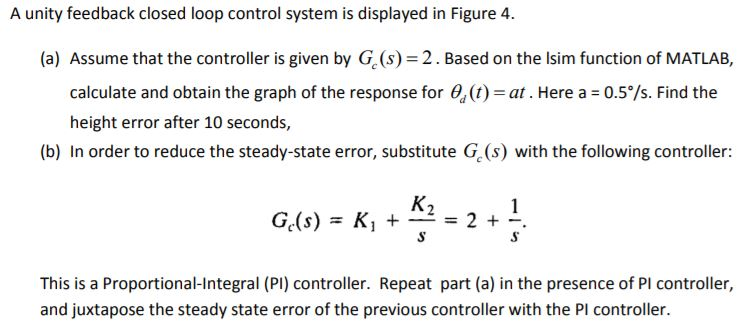Please solve as a MATLAB code. A unity feedback closed loop control system is displayed in Figure 4. (a) Assume that the controller is given by G (s) 2. Based on the lsim function of MATLAB, calculate and obtain the graph of the response for (t) at. Here a 0.5°/s. Find the height error after 10 seconds, (b) In order to reduce the steady-state error, substitute G (s) with the following controller This is a Proportional-Integral (PI) controller. Repeat part...

• ### Problem 1: Steady-state error analvsis (a) A block diagram of a feedback control system is given ...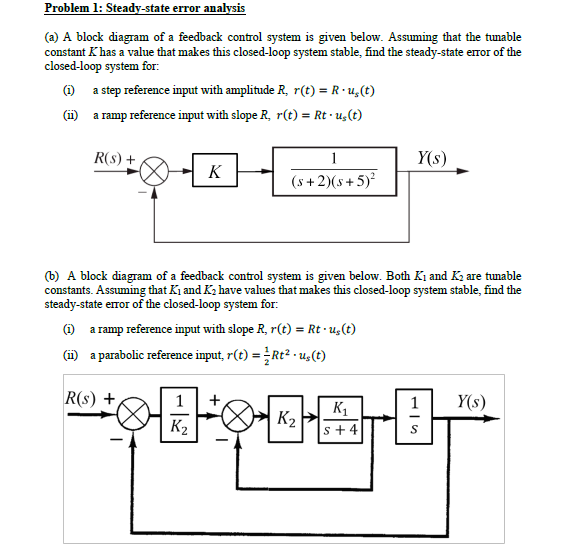The Class Name is: MAE 318 System Dynamics and Control I Problem 1: Steady-state error analvsis (a) A block diagram of a feedback control system is given below. Assuming that the tunable constant Khas a value that makes this closed-loop system stable, find the steady-state error of the closed-loop system for (a a step reference input with amplitude R, r(t)- R u(t) (ii) a ramp reference input with slope R, r(t) = Rt-us(t) R(s) Y(s) (s+2)(s +5) (b) A block...

• ### A unity feedback system is shown in Fig. 1. The closed-loop transfer function ?(?) of this...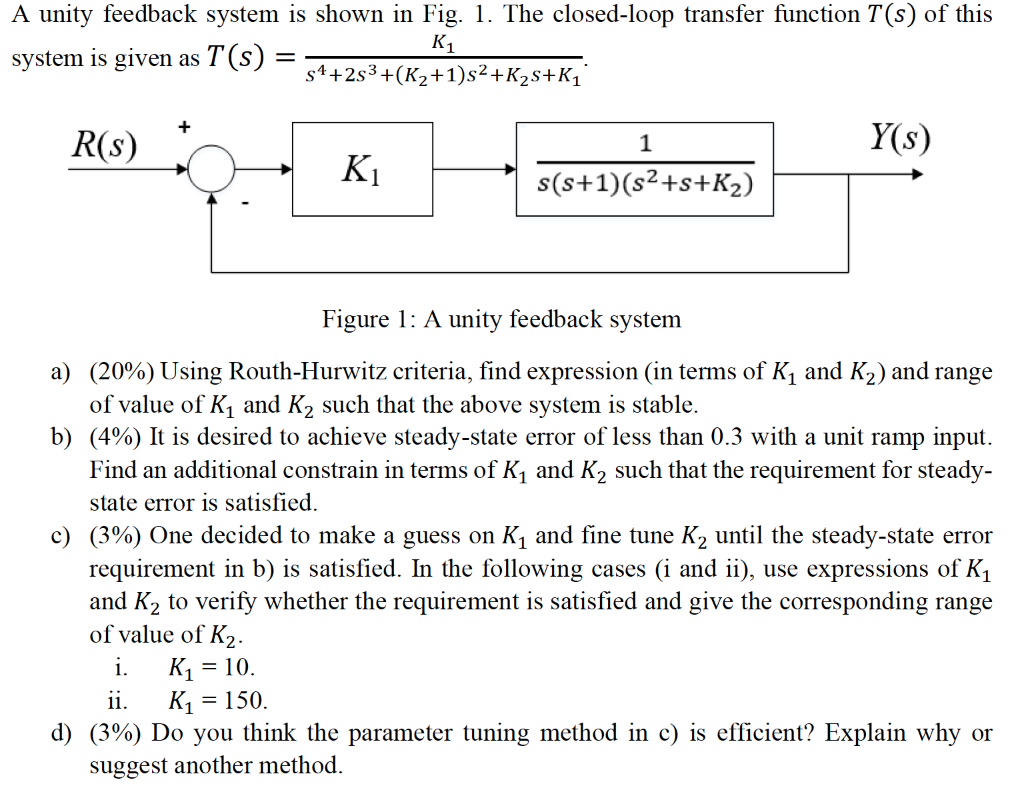A unity feedback system is shown in Fig. 1. The closed-loop transfer function ?(?) of this system is given as ?(?)=?1?4+2?3+(?2+1)?2+?2?+?1. a) (20%) Using Routh-Hurwitz criteria, find expression (in terms of ?1 and ?2) and range of value of ?1 and ?2 such that the above system is stable. b) (4%) It is desired to achieve steady-state error of less than 0.3 with a unit ramp input. Find an additional constrain in terms of ?1 and ?2 such that the...

• ### plz solve this problem  Consider the system shown below. Design the PD controller such that the closed loop syst...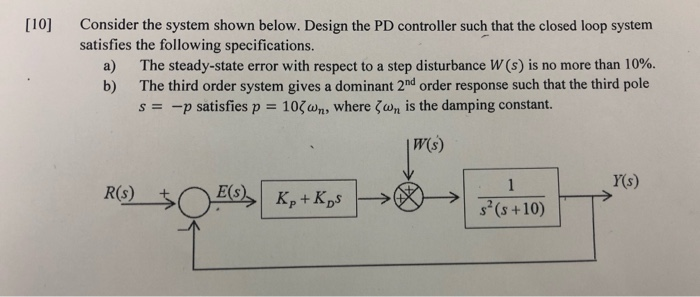plz solve this problem  Consider the system shown below. Design the PD controller such that the closed loop system satisfies the following specifications. a) The steady-state error with respect to a step disturbance W (s) is no more than 10 %. b) The third order system gives a dominant 2nd order response such that the third pole s=p satisfies p 10wn, where Zwn is the damping constant. |W(s) Y(s) 1 E(S)Kp+Kps R(s) s(s+10)  Consider the system shown below....

• ### blem 5 (2000): The closed-loop system is given below. Controller El(s) ) (5% o) Find the system transfer function and discuss the range of Ko to make the stem stable assuming Kp-5. ) (5 %) Find t...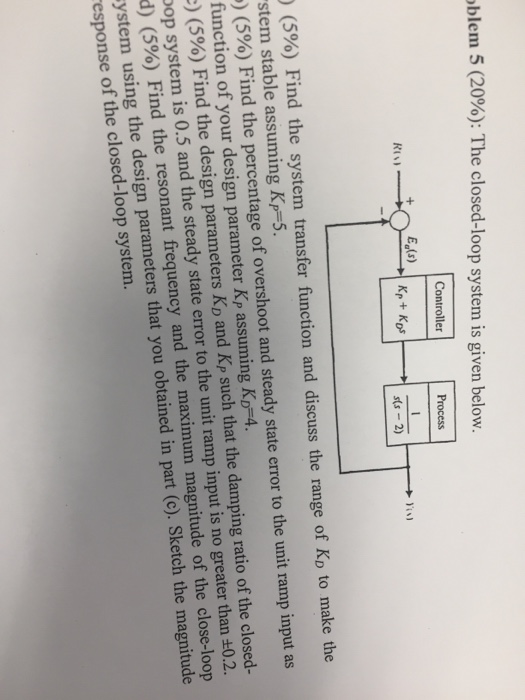blem 5 (2000): The closed-loop system is given below. Controller El(s) ) (5% o) Find the system transfer function and discuss the range of Ko to make the stem stable assuming Kp-5. ) (5 %) Find the percentage of overshoot and steady state error to the unit ramp input as function of your design parameter Kp assuming KD-4. :) (5%) Find the design parameters KD and Kp such that the damping ratio of the closed- pop system is 0.5 and...

• ### PROBLEMA: (25%) A closed-loop control system is shown below Ds) T(O) U(A) C(s) (a) Show that a proportional controller (C(s)-kp) will never make the closed-loop system stable. (8%) (Hint: you nee...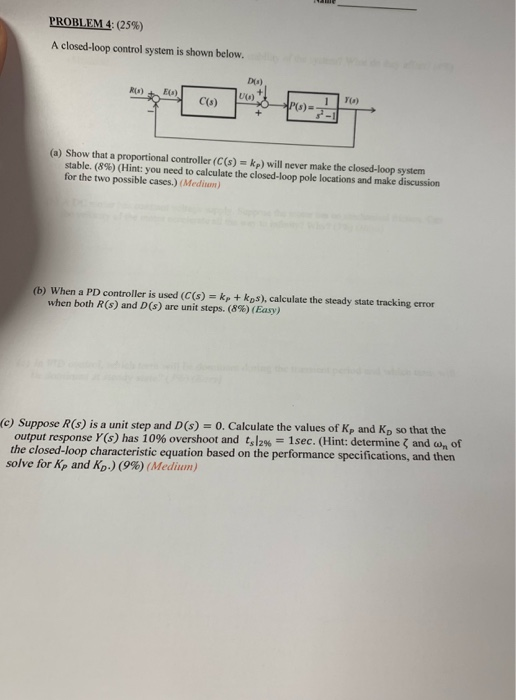PROBLEMA: (25%) A closed-loop control system is shown below Ds) T(O) U(A) C(s) (a) Show that a proportional controller (C(s)-kp) will never make the closed-loop system stable. (8%) (Hint: you need to calculate the closed-loop pole locations and make discussion for the two possible cases.) (Medim) (b) When a PD controller is used (C(s)kp+ kps), calculate the steady state tracking error when both R(s) and D(s) are unit steps. (8%) (Easy) (e) Suppose R(s) is a unit step and D(s)...